Chapter2：Configuration Space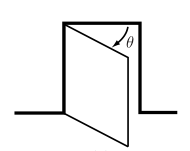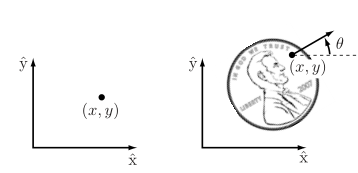Definition 1： The configuration of a robot is a complete specification of the position of every point of the robot. The minimum number n of real-valued coordinates needed to represent the configuration is the number of degrees of freedom (dof) of the robot. The n-dimensional space containing all possible configurations of the robot is called the configuration space (C-space). The configuration of a robot is represented by a point in its C-space.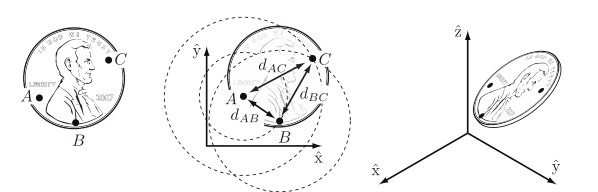degrees of freedom =(sum of freedoms of the points)-

(number of independent constraints)

degrees of freedom = (number of variables)-(number of independent equations)

degrees of freedom = (sum of freedoms of the bodies) -

(number of independent constraints)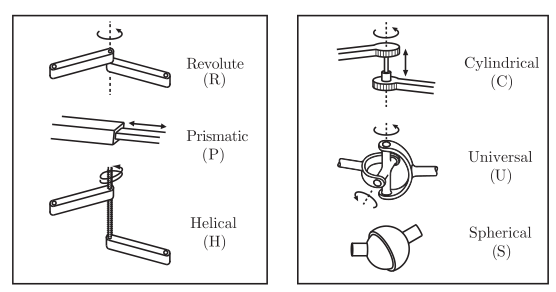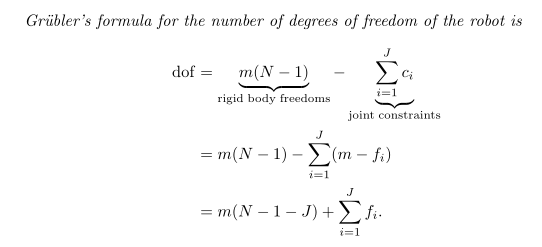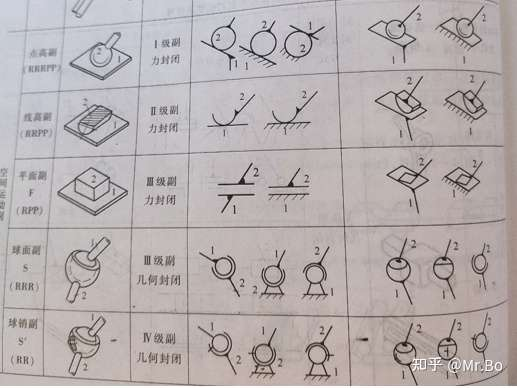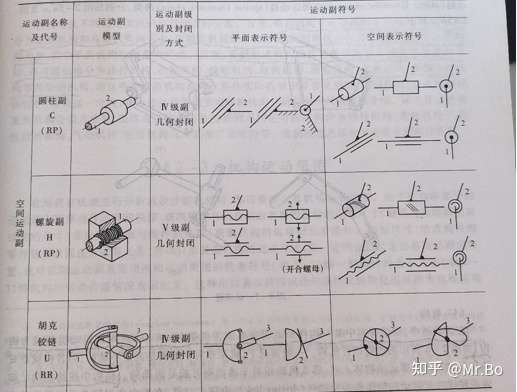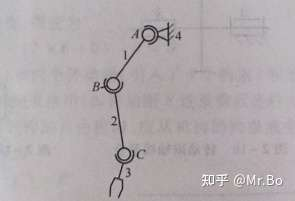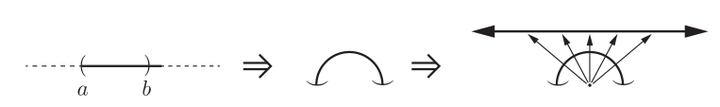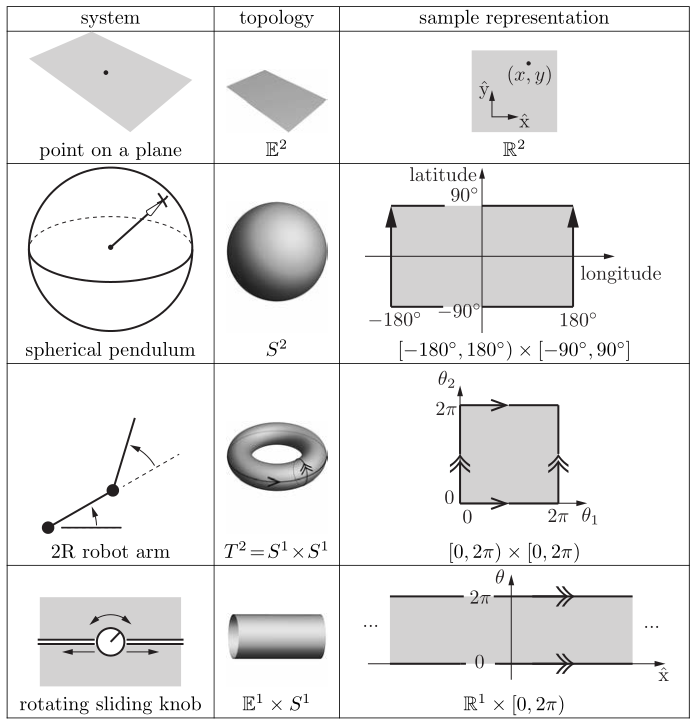（1）在空间上使用多个坐标图，其中每个坐标图都是只覆盖空间的一部分的显式参数化，这样在每个图中就没有奇点。

（2）使用隐式表示，而不是显式参数化。隐式表示法将n维空间视为嵌入在超过n维的欧几里德空间中，就像一个二维单位球可以被视为嵌入在三维欧几里德空间中的表面一样。在之后的各个章节中将使用隐式表示。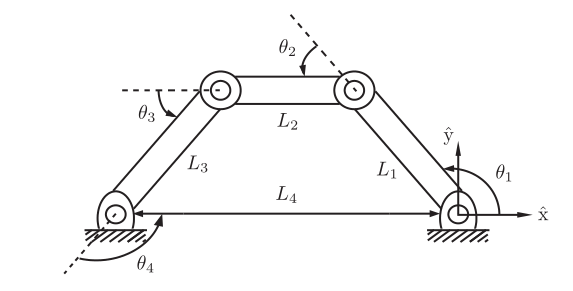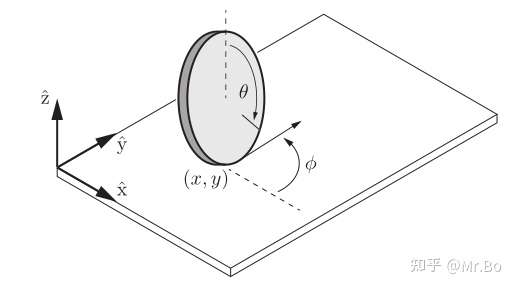The task space is a space in which the robot’s task can be naturally expressed.

The workspace is a specification of the configurations that the end-effector of the robot can reach.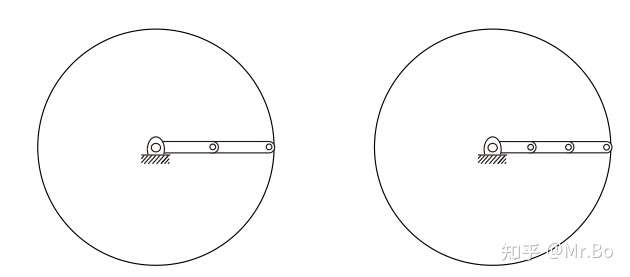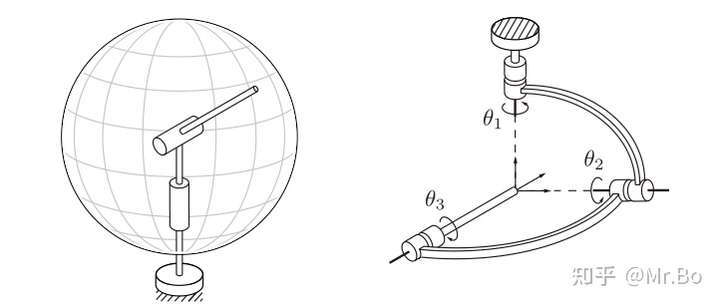chapter2的内容结束，原书中有些例子省略了，感兴趣可以去看看